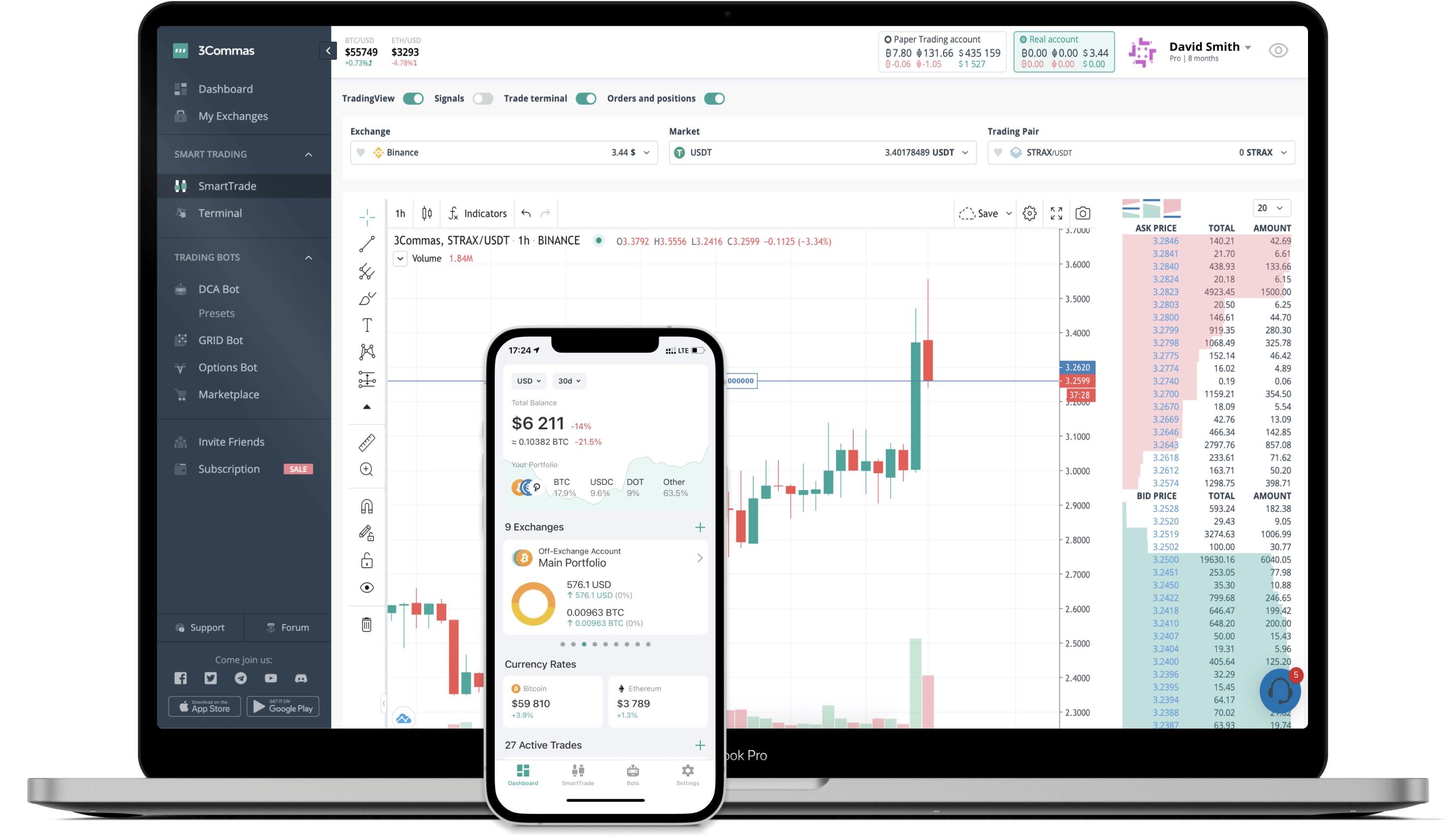# MATH to BRL Converter

MATH
PriceBRL 4.61+0.73%
VolumeBRL 8,204,229
How it works

The 3Commas currency calculator allows you to convert a currency from MATH (MATH) to Brazilian Real (BRL) in just a few clicks at live exchange rates.

Simply enter the amount of MATH you wish to convert to BRL and the conversion amount automatically populates. You can also use our Prices Calculator Table to calculate how much your currency is worth in other denominations, i.e. .1 MATH, .5 MATH, 1 MATH, 5 MATH, or even 10 MATH.

## MATH to BRL Exchange Calculator

The chart displays MATH price changes in BRL.

The ultimate tools for crypto traders to maximize trading profits while minimizing risk and loss.## Latest MATH to BRL price calculator

MATH market price is updated every three minutes and is automatically displayed in BRL. Below are the most popular denominations to convert to BRL.

MATH
Brazilian Real
0.01
MATH
0.05
BRL
0.1
MATH
0.46
BRL
1
MATH
4.61
BRL
2
MATH
9.22
BRL
3
MATH
13.83
BRL
5
MATH
23.05
BRL
10
MATH
46.11
BRL
25
MATH
115.27
BRL
50
MATH
230.54
BRL
100
MATH
461.08
BRL
250
MATH
1152.70
BRL
500
MATH
2305.40
BRL
1000
MATH
4610.80
BRL
2500
MATH
11527.00
BRL
Brazilian Real
MATH
0.01
BRL
0.00216882
MATH
0.1
BRL
0.02168821
MATH
1
BRL
0.21688212
MATH
2
BRL
0.43376424
MATH
3
BRL
0.65064636
MATH
5
BRL
1.08441060
MATH
10
BRL
2.16882121
MATH
25
BRL
5.42205301
MATH
50
BRL
10.84410603
MATH
100
BRL
21.68821206
MATH
250
BRL
54.22053014
MATH
500
BRL
108.44106029
MATH
1000
BRL
216.88212057
MATH
2500
BRL
542.20530143
MATH

## FAQ

### How much is 1 MATH in Brazilian Real?

MATH price in BRL is constantly changing.

At this moment, 1 MATH equals 4.610799624061456 BRL

### How do I calculate the conversion from MATH to BRL?

The 3Commas MATH Calculator allows you to easily calculate the conversion price of MATH to BRL by simply entering the amount of MATH in the corresponding field and will automatically convert the value in Brazilian Real (BRL).

You can also use our MATH price table above to check the latest MATH price in major fiat and crypto currencies.

### How can I convert MATH to BRL?

The most common way of converting MATH to BRL is by using a Crypto Exchange or a P2P (person-to-person) exchange platform like LocalBitcoins, etc.